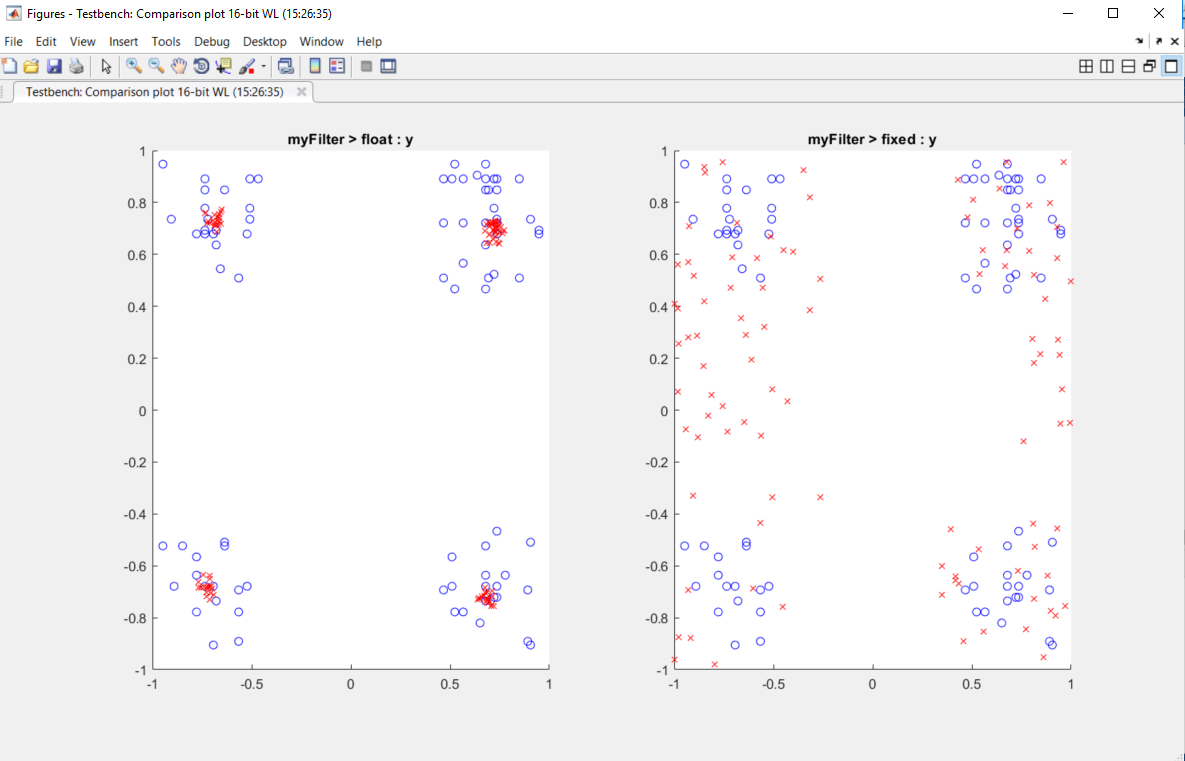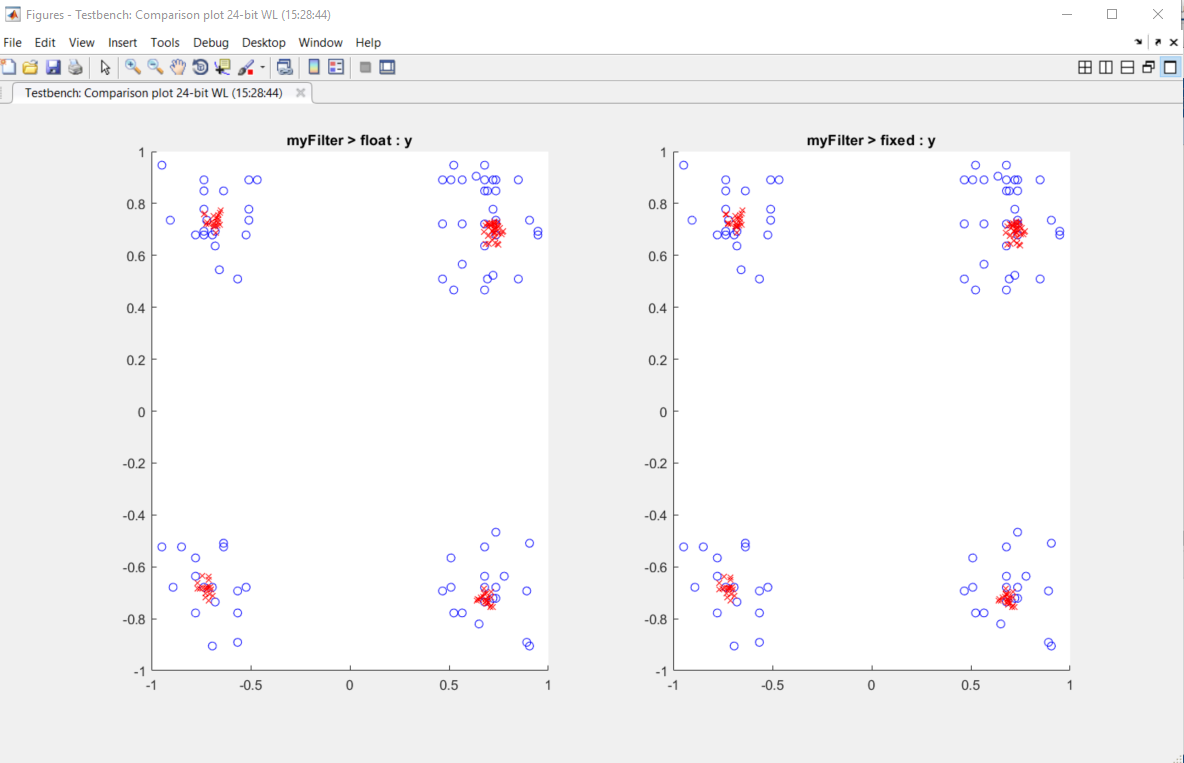## Visualize Differences Between Floating-Point and Fixed-Point Results

This example shows how to configure the `fiaccel` function to use a custom plot function to compare the behavior of the generated fixed-point code against the behavior of the original floating-point MATLAB® code.

By default, when the `LogIOForComparisonPlotting` option is enabled, the conversion process uses a time series based plotting function to show the floating-point and fixed-point results and the difference between them. However, during fixed-point conversion you might want to visualize the numerical differences in a view that is more suitable for your application domain. This example shows how to customize plotting and produce scatter plots at the test numerics step of the fixed-point conversion.

### Prerequisites

To complete this example, you must install the following products:

• MATLAB

• Fixed-Point Designer™

• C compiler

You can use `mex -setup` to change the default compiler. See Change Default Compiler.

### Create a New Folder and Copy Relevant Files

1. Create a local working folder, for example, `c:\custom_plot`.

2. Change to the `docroot\toolbox\fixpoint\examples` folder. At the MATLAB command line, enter:

`cd(fullfile(docroot, 'toolbox', 'fixpoint', 'examples')) `

3. Copy the `myFilter.m`, `myFilterTest.m`, `plotDiff.m`, and `filterData.mat` files to your local working folder.

It is best practice to create a separate test script to do all the pre- and post-processing such as loading inputs, setting up input values, calling the function under test, and outputting test results.

TypeNameDescription
Function code`myFilter.m`Entry-point MATLAB function
Test file`myFilterTest.m`MATLAB script that tests `myFilter.m`
Plotting function`plotDiff.m`Custom plot function
MAT-file`filterData.mat`Data to filter.

### Set Up Configuration Object

1. Create a `coder.FixptConfig` object.

`fxptcfg = coder.config('fixpt');`
2. Specify the test file name and custom plot function name. Enable logging and numerics testing.

```fxptcfg.TestBenchName = 'myFilterTest'; fxptcfg.PlotFunction = 'plotDiff'; fxptcfg.TestNumerics = true; fxptcfg. LogIOForComparisonPlotting = true; fxptcfg.DefaultWordLength = 16; ```

### Convert to Fixed Point

Convert the floating-point MATLAB function, `myFilter`, to fixed-point MATLAB code. You do not need to specify input types for the `fiaccel` command because it infers the types from the test file.

`fiaccel -args {complex(0, 0)} -float2fixed fxptcfg myFilter`

The conversion process generates fixed-point code using a default word length of `16` and then runs a fixed-point simulation by running the `myFilterTest.m` function and calling the fixed-point version of `myFilter.m`.

Because you selected to log inputs and outputs for comparison plots and to use the custom plotting function, `plotDiff.m`, for these plots, the conversion process uses this function to generate the comparison plot.The plot shows that the fixed-point results do not closely match the floating-point results.

Increase the word length to `24` and then convert to fixed point again.

```fxptcfg.DefaultWordLength = 24; fiaccel -args {complex(0, 0)} -float2fixed fxptcfg myFilter```

The increased word length improved the results. This time, the plot shows that the fixed-point results match the floating-point results.## SupportGet trial now